Students can access the CBSE Sample Papers for Class 12 Chemistry with Solutions and marking scheme Term 2 Set 8 will help students in understanding the difficulty level of the exam.

## CBSE Sample Papers for Class 12 Chemistry Term 2 Set 8 with Solutions

Time Allowed: 2 Hours
Maximum Marks: 35

General Instructions:

• There are 12 questions in this question paper with internal choice.
• SECTION A – Q. No. 1 to 3 are very short answer questions carrying 2 marks each.
• SECTION B – Q. No. 4 to 11 are short answer questions carrying 3 marks each.
• SECTION C- Q. No. 12 is case based question carrying 5 marks.
• All questions are compulsory.
• Use of log tables and calculators is not allowed.

Section – A
(Section A-Question No 1 to 3 are very short answer questions carrying 2 marks each.)

Question 1.
What is meant by the following terms?
(i) Cyanohydrin
-OH group and cyano group are present on same carbon atom. Addition of HCN to carbonyl group in weakly acidic medium forms cyanohydrin.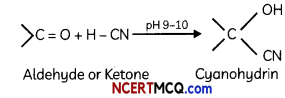(ii) Acetal (2)
Acetal : Terminal C atom has two alkoxy groups. Two equivalents of monohydric alcohol add to 1 equivalent of aldehyde in presence of dry HCl gas.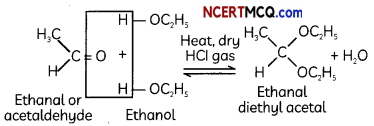Caution:
Students should draw the related structures to get full marks.Question 2.
Arrange the following as instructed: (Any two)
(A) In an increasing order of basic strength CH6H5NH2, C6H5N(CH3)2 (C2H6)2 NH and CH3 NH2.
C6H5NH2 < C6H5N(CH3)2 < CH3NH2 < (C2H5)62NH.

Explanation:
In C6H5N(CH3)2 presence of two CH3 groups (increases the electron density of N-atom) makes it . more basic than C6H5NH3 but less basic than (C2H5)2NH2 and CH3NH2 due to the presence of aromatic ring which is responsible for the delocalisation of lone pair of electrons of N-atom over the benzene ring (decreases the electron density of N-atom).

(B) In a decreasing order of basic strength Aniline, p-nitroaniline and p-toluidine.
p-nitro aniline < Aniline < p-toluidine

Explanation:
In p-toluidine, the presence of electron-donating-CH3 group increases the electron density on the N-atom.
Thus, p-toluidine is more basic than aniline. On the other hand, the presence of electron – withdrawing – NO2 group decreases the electron density over the N-atom in p-nitroaniline. Thus, p-nitroaniline is less basic than aniline.

(C) In an increasing order of pKb values
C2H5NH2, C6H5NHCH3, (C2H5)2 NH and C6H5NH2 (2)
(C2H5)2 NH < C2H5NH2 < C6H5NHCH3 < C6H5NH2.

Explanation:
In C2H5NH2, only one -C2H5 group is present while in (C2H5)22NH, two – C2H5 groups are present. Thus, the +1 effect is more in (C2H5)2NH than in C2H5NH2. Therefore, the electron density over the N-atom is more in (C2H5)2NH than in C2H5NH2. Hence, (C2H5)2NH is more basic than C2H5NH2.

Also, both C6H5NHCH3 and C6H5NH2 are less basic than (C2H5)2NH and C2H5NH2 due to the delocalization of the lone pair in the former two. Further, among C6H5NHCH3 and C6H5NH2, the former will be more basic due to the +1 effect of -CH3 group.

Question 3.
What is meant by rate of a reaction? Differentiate between average rate and instantaneous rate of a reaction. (2)
Rate of a chemical reaction is the change in the concentration of any one of the reactants or products per unit time. It is expressed in mol L-1 s-1 or Ms-1 or atm time-1 units.

The average rate of a reaction is defined as the rate of change of concentration of a reactant (or of a product) over a specified measurable period of time.
Instantaneous rate of reaction gives the tendency of the reaction at a particular point of time during its course (or) The time derivative of the concentration of a reactant (or product) converted to a positive number is called the instantaneous rate of reaction.

Rate of disappearance of RSection – B
(Section B-Question No 4 to 11 are short answer questions carrying 3 marks each.)

Question 4.
Write the IUPAC names of the following coordination compounds:
(i) [Co(NH3)6]Cl3
(ii) [Cr(NH3)5Cl]Cl2
(iii) K3[Fe(CN)6]
OR
Explain different types of ligands with one example of each. (3)
(i) Hexaamminecobalt (III) chloride.
(ii) Pentaamminechloridochromium (III) chloride.
(iii) Potassium hexacyanidoferrate (III).
OR
The ions or molecules bound to central atom or ion in the coordination entity are ligands e.g. [Fe(CN)6]4- has six CN- ligands.

Types:

1. On the basis of charges on them ligands can be negative, positive (e.g. H3O+. NH4+ etc) or neutral (eg. CO, NH3, H2O).
2. On the basis of their donor atoms ligands can be monodentate or unidentate (one donor atom) e.g- NH3, H2O, Cl- etc or didentgte (two donor atoms) H2NCH2CH2NH2 or C2O42- etc or polydentate (several donor atoms) eg. [EDTA]4- is a hexadentate ligand.
3. Ligands which can ligate through two different atoms are called ambidentate ligands eg. NO2 and SCN ions whereas when a di- or polydentate ligand uses its two or more donor atoms to bind a single metal ion, it is called chelate ligand.

Question 5.
The conductivity of 0.001 mol L-1 solution of CH3COOH is 3.905 × 10-5 S cm-1. Calculate its molar conductivity and degree of dissociation (a).
Given: λ°(H+) = 349.6 S cm2 mol-1 and λ° (CH3COO) = 40.9 S cm2 mol-1 (3)
5. C = 0.001 mol L-1, k = 3.905 x 10-5 S cm-1

Molar conductivity
K = 3.905 × 10-5 S cm-1cm = ?

Using formula,
cm = $$\frac{K \times 1000}{C}=\frac{3.905 \times 10^{-5} \times 1000}{0.001}$$
=39.05 S cm2 mol-1

Molar conductivity at infinite dilution:
CH3COOH → CH3COC + H+
∧°m = λ°H++ λ°CH3COO
= (349.6 + 40.9)
∧°m = 390.5 S cm2 mol-1

Degree of dissociation:
α = $$\frac{\wedge^{c} \mathrm{~m}}{\wedge^{\circ} \mathrm{m}}=\frac{39.05}{390.5}$$
∴α = 0.1

Question 6.
A first order gas phase reaction : A2B2(g) → 2A(g) + 2B(g) at the temperature 400°C
has the rate constant k = 2.0 × 10-4 sec-1. What percentage of A2B2 is decomposed on heating for 900 seconds? (Antilog 0.0781 = 1.197).
OR
The thermal decomposition of HC02H is a first order reaction with a rate constant of 2.4 × 10-3 s-1 at a certain temperature. Calculate how long will it take for three-fourths of initial quantity of HCOOH to decompose, (log 0.25 = -0.6021) (3)
Since the reaction is of the first order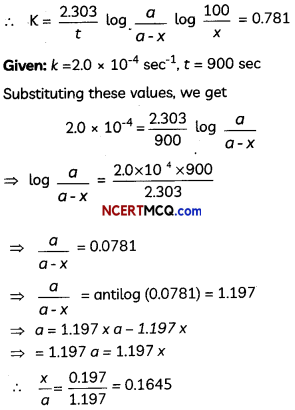OR
Given : K = 2.4 × 10-4 sec-1Question 7.
(A) Write the electronic configuration of Ce3+ ion, and calculate the magnetic moment on the basis of the ‘spin-only’ formula. [Atomic No. of Ce = 58]
Ce(58) : [Xe]4 f45d16s2
Ce3+(58): [Xe]4 f1

Spin only Formula
Magentic moments= 1.732 B.M.
where ‘S’ is spin quantum number, Ce3+ has one unpaired electron which has spin quantum number equal to 1/2.

(B) Account for the following:
(i) The entholpies of atomisation of the transition metals are high.
It is because of strong metallic bonds due to large number of unpaired electrons in d-orbitals.

Explanation:
The enthalpies of atomization of the transition metals are high because of large number of unpaired electrons in their atoms, they have stronger inter atomic interaction and hence strong metallic bonding is present between atoms.

(ii) The lowest oxide of o transition metal is basic, the highest is amphoteric/ acidic. (3)
It is because transition metals in lowest oxidation state are more metallic and in higher oxidation state are least metallic, therefore, oxides in Lower oxidation state are basic, whereas in higher oxidation state are amphoteric/acidic

Explanation:
In Low oxidation state of the metaL, some of the valence electrons of the metal atom are not involved in bonding. Hence it can donate electrons and behave as a base. On the other hand, in higher oxidation state of the metal, valence electrons are involved in bonding and are not available. Instead effective nuclear charge is high and hence it can accept electrons and behave as an acid.

Question 8.
(A) Arrange the following complex ions in increasing order of crystal field splitting energy ():
[Cr(Cl)6]3-, [Cr(CN)6]3-, [Cr(NH3)6]3+.
Crystal field splitting energy AO increases
in the order:
[Cr(Cl)6]3- < [Cr(NH3)6]3+ < [Cr(CN)6]3-

Explanation:
Among the ligands Cl, NH3 and CN, Cl is the weakest ligand and CN is the strongest one. Therefore, increasing order of crystal field splitting energy A0 is:
[Cr(Cl)6]3- < [Cr(NH3)6]3+ < [Cr(CN)6]3-

(B) Why do compounds having similar geometry have different magnetic moments?
Strong field ligand can easily cause the pairing of electrons while the weak field Ligands are not able to form pairs. The magnetic moment of a compound depends on the number of unpaired or paired electrons. Hence it is different for compounds having similar geometry.
Explanation – If CFSE is high, the complex will show low value of magnetic moment and vice versa, e.g. [CoF6]3- and [Co(NH3)6]3+, the former is paramagnetic and the latter is diamagnetic.

(C) Name the type of Isomerism when ambidentate Ligands are attached to o central metal ion. Give one example this isomerism. (3)
Linkage Isomerism is a type of isomerism when ambidentate ligands get attached to central metal ions.

Examples:
[Co(NH3)5ONO]Cl2(Pentaammine nitrito cobalt (III) chloride) – O – attached. (Red in colour) and [CO(NH3)5NO2]Cl2 (Pentaammine nitro cobalt (III) chloride) – N – attached (Yellow-brown in colour)

Related Theory:
The Ligands which have two binding sites are known as Ambidendate ligands. Some of the examples are: Nitrite-N, Nitrito-0 and Isothidcyanato, Thiocyanato.

Question 9.
Predict the products in the followng reactions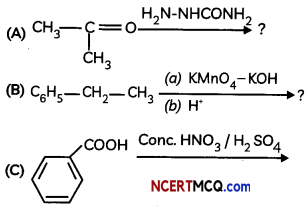OR
Two motes of compound (A) on treatment with a strong base gives two compounds (B) and (C). The compound (B) on dehydrogenation with Cu gives (A) white acidification of (C) gives carboxytic acid (D) having molecular formulo CH2O2. Identify (A) to (D). (3)Since (D) is a carboxylic acid with one carbon only, it is HCOOH. As it is obtained from (C) acidification, (C) COONa and (A) is HCHO which on treatment with strong base (NaOH) gives CH3OH & HCOONa (Cannizaro’s reaction).

The reactions are: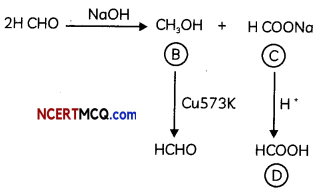Question 10.
How would you account for the following:
(A) Etectrophitic susbstitution ¡n case of aromatic amines takes place more readily than benzene.
(B) Ethanamide is a weaker base than ethonamine.
(C) It is difficult to prepare pure amines by ammonotysis of atkyl halides. (3)
OR
Give the structures of A, B and C in the following reactions: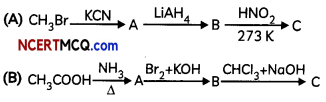(A) Due to the strong activating effect (+1) of the – NH2 group, aromatic amines undergo electrophilic substitution reactions readiLy than benzene.

(B) In case of acetamide due to resonance, the lone pair of electrons on the nitrogen atom is delocalized over keto group which decreases electron density hence less basic while in ethylamine due to +1 effect of ethyl group electron density increases on N-atom and hence basic character increases.

(C) By ammonolysis of alkyl halides, a mixture of primary, secondary and tertiary amines is formed. The separation of these amines is very diffcult.
Thus, it is very difficult to prepare pure amines by ammonolysis of alkyl halides.
OR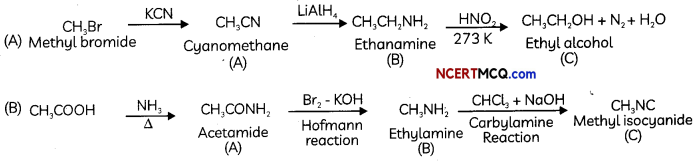Question 11.
(A) Benzaldehyde can be obtained from benzol chloride. Write reactions for obtaining benzol chloride and then benzaldehyde from it.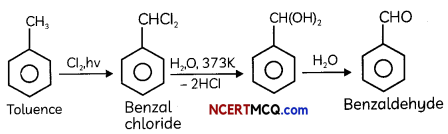Explanation:
It is the commercial method for preparing benzaldehyde. Benzal chloride can be obtained by photochlorination of toluene, i.e. chlorination of toluene in the presence of sunlight. Then, benzal chloride on heating with boiling water produces benzaldehyde.

(B) Name the electrophile produced in the reaction of benzene with benzoyt chloride in the presence of anhydrous AlCl3. Name the reaction also.
The electrophile produced in the reaction of benzene with benzoyl chloride in the presence of anhydrous AlCl3 is benzoylinium cation (C6H5CO+). The product formed in this reaction is benzophenone. This reaction is called Friedel Craft’s acylation or benzoylation reaction.

Explanation: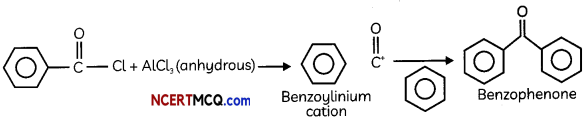(C) Give the structure of the following compound – 4-Nitro Propiophenone (3)
4-Nitro Propiophenone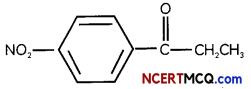Section – C
(Section C-Question No 12 is case-based question carrying 5 marks.)

Question 12.
Hardly Schulze rule states that the precipitating effect of an ion on dispersed phase of opposite charge increases with the valency of the ion. The higher the valency of the flocculating ion, the greater is its precipitating power. Thus, for the precipitation of AS2S3 sol (-ve sol) the precipitating power of Al3+ > Ba2+ > Na and Na+ ion is of the orde Al3+ > Na2+ Na+. Similarly, for precipitating Fe(OH)3 sol (+ ve sol) the precipitating power of [Fe(CN)6]3-, SO442- and Cl is of the order, [Fe(CN)6]3- > <SO42- > Cl. The minimum concentration of an electrolyte in million per litre required to cause precipitation of a sol in 2 hours is called flocculation value.

The smaller the flocculation value, the higher will be the coagulating power of the ion. The minimum mass of the protective colloid (lyophilic colloid) in milligrams that must be added to 10 ml. of a standard red gold sol so that no coagulation occurs when I ml. of 10% NaCl solution is rapidly added to it is called the gold number of the protective colloid.

(A) Arrange the following ions in the increasing order of their flocculation value:
Cl, P043-, S042-, [Fe(CN)6]4-
(B) What is gold number?
(C) State Hardy Schulze rule.
(D) (i) Out of AlCl3 and KCl, which is more effective in causing coagulation of a negative sol and why?
(ii) What happens when an electrolyte is added to a hydrated ferric oxide sol in water and why?
OR
Explain the following:
(i) Deltas are formed when river and seawater meet.
(ii) Artificial rain is caused by spraying salt over clouds. (5)
(A) [Fe(CN)6]4- < P043- < S042- < Cl

Explanation:
More the charge on flocculating ion, less the flocculation value.

(B) The gold number is defined as the minimum mass of the colloid in milligram that is added to the 10ml of red gold sol to protect it from the coagulation when 1 ml of 10% NaCl is added.

(C) Hardy Schulze rule states that the amount of electrolyte required for the coagulation of a definite amount of a colloidal solution is dependent on the valency of the coagulating ion. Coagulating ion is the ion which has the charge opposite to the charge of the colloidat particles).

(D) (i) AlCl3, because Al3+ has higher charge than K+ ion. Higher the charge, more effective it will be for coagulation. Explanation: The greater the magnitude of the opposite charge, the higher the ability of a salt to coagulate the sol. Thus, trivalent salt AlCl3 is more effective in causing the coagulation of a negatively charged sol than monovalent salt KCl.

(ii) The positively charged colloidal particles of Fe(OH)3 get coagulated by the negatively charged ions provided by electrolyte. Explanation: When an electrolyte like KCl is added of Fe(OH)3 sol, the positively charged colloidal particles of Fe(OH)3 get coagulated by the oppositely charged Cl ions provided by KCl.
OR
(D) (i) River water is a colloidal solution of clay. Sea water contains a number of electrolytes. When river water meets the sea water, the electrolytes present in sea water coagulate the colloidal solution of clay resulting in its deposition with the formation of deltas.

Explanation:
River water is the negatively charged colloidal solution of clay and sands, whereas sea water contains a number of electrolytes. At the meeting point of sea water and river water, the electrolytes present in sea water neutralize and coagulate the colloidal solution of clay and sand resulting in their deposition leading to the formation of delta.

(ii) Clouds are colloidal dispersion of water particles in air carrying some charge over them.
It is possible to cause artificial rain by throwing electrified sand or spraying a sol carrying charge opposite to the one on clouds from an aeroplane. The colloidal water particles present in the clouds will get neutralized and as result they will come closer and grow in size to form bigger water drops and ultimately cause artificial rain.